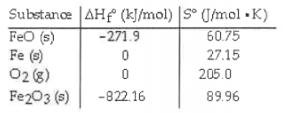# Problem: Consider the reaction: FeO(s) + Fe(s) + O2(g)→ Fe2O3(s) Given the following table of thermodynamic data, determine the temperature (in C°) which the reaction is nonspontaneous. a. This reaction is spontaneous at all temperatures. b. 6180.1 c. 756.3 d. 2438 e. 1235

###### FREE Expert Solution
94% (181 ratings)
###### Problem Details

Consider the reaction:

FeO(s) + Fe(s) + O2(g)→ Fe2O3(s)

Given the following table of thermodynamic data, determine the temperature (in C°) which the reaction is nonspontaneous.

a. This reaction is spontaneous at all temperatures.

b. 6180.1

c. 756.3

d. 2438

e. 1235What scientific concept do you need to know in order to solve this problem?

Our tutors have indicated that to solve this problem you will need to apply the Spontaneous Reaction concept. You can view video lessons to learn Spontaneous Reaction. Or if you need more Spontaneous Reaction practice, you can also practice Spontaneous Reaction practice problems.

What is the difficulty of this problem?

Our tutors rated the difficulty ofConsider the reaction: FeO(s) + Fe(s) + O2(g)→ Fe2O3(s) Gi...as high difficulty.

How long does this problem take to solve?

Our expert Chemistry tutor, Dasha took 13 minutes and 19 seconds to solve this problem. You can follow their steps in the video explanation above.

What professor is this problem relevant for?

Based on our data, we think this problem is relevant for Professor Griffith's class at UMD.